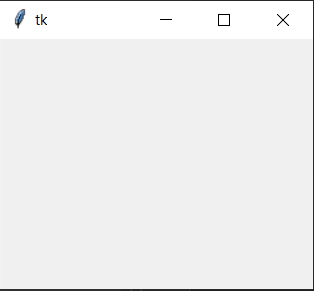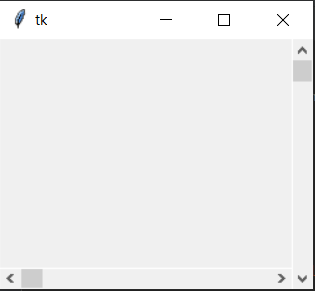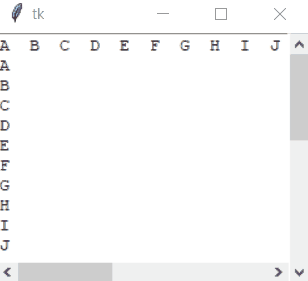# How to make a proper double scrollbar frame in Tkinter

Tkinter is a Python binding to the Tk GUI(Graphical User Interface) Toolkit. It is a thin-object oriented layer on top of Tcl/Tk. When combined with Python, it helps create fast and efficient GUI applications.

#### Steps to Create a double scrollbar frame in Tkinter

1) Firstly, the module Tkinter will be imported as:

`import tkinter as tk `

So, tkinter is abbreviated here as tk so as to make the code look cleaner and efficient.

Now, a window will be created to display:

 `import` `tkinter as tk  ` ` `  ` `  `window ``=` `tk.Tk() ` `window.geometry(``"250x200"``) `

Output:Functions to understand:

• geometry(): This method is used to set the dimensions of the Tkinter window as well as it is used to set the position of the main window on the user’s desktop.

2) The next code is to assign to the scrollbars for horizontal and vertical.

 `SVBar ``=` `tk.Scrollbar(window) ` `SVBar.pack (side ``=` `tk.RIGHT, ` `            ``fill ``=` `"y"``) ` ` `  `SHBar ``=` `tk.Scrollbar(window,  ` `                     ``orient ``=` `tk.HORIZONTAL) ` `SHBar.pack (side ``=` `tk.BOTTOM,  ` `            ``fill ``=` `"x"``) `

Output:Functions to understand:

• Scrollbar() = It is the scrollbar that is allotted to the sides of the window.
• pack() method: It organizes the widgets in blocks before placing in the parent widget.

3) Now, make a text box for the window:

 `TBox ``=` `tk.Text(window, ` `               ``height ``=` `500``, ` `               ``width ``=` `500``, ` `               ``yscrollcommand ``=` `SVBar.``set``, ` `               ``xscrollcommand ``=` `SHBar.``set``,  ` `               ``wrap ``=` `"none"``) ` ` `  `TBox ``=` `tk.Text(window,  ` `               ``height ``=` `500``, ` `               ``width ``=` `500``,  ` `               ``yscrollcommand ``=` `SVBar.``set``,  ` `               ``xscrollcommand ``=` `SHBar.``set``,  ` `               ``wrap ``=` `"none"``) ` ` `  `TBox.pack(expand ``=` `0``, fill ``=` `tk.BOTH) `

Functions to understand:

• Text() = It is a textbox widget of a standard Tkinter widget used to display the text.
• pack() = It is a geometry manager for organizing the widgets in blocks before placing them in the parent widget. Options like fill, expand and side are used in the function.
```SHBar.config(command = TBox.xview)
SVBar.config(command = TBox.yview)```

Here, within the arguments of the function `config()`, the scrollbars are assigned at their specific x-axis and y-axis and are able to function.

Now, insert some amount of text to display:

Num_Vertical = (“\nA\nB\nC\nD\nE\nF\nG\nH\nI\nJ\nK\nL\nM\nN\nO\nP\nQ\nR\nS\nT\nU\nV\nW\nX\nY\nZ”)
Num_Horizontal = (“A B C D E F G H I J K L M N O P Q R S T U V W X Y Z”)

To insert the text in the window for the display, the following code is done:

 `TBox.insert(tk.END, Num_Horizontal) ` `TBox.insert(tk.END, Num_Vertical) `

Complete Code:

 `import` `tkinter as tk ` `  `  ` `  `Num_Vertical ``=` `("\nA\nB\nC\nD\nE\nF\nG\n\ ` `H\nI\nJ\nK\nL\nM\nN\nO\nP\nQ\nR\nS\nT\n\ ` `U\nV\nW\nX\nY\nZ") ` `Num_Horizontal ``=` `("A  B  C  D  E  F  G  H \ ` `I  J  K  L  M  N  O  P  Q  R  S  T  U  V \ ` `W  X  Y  Z") ` `  `  `window ``=` `tk.Tk() ` `window.geometry(``"250x200"``) ` `  `  `SVBar ``=` `tk.Scrollbar(window) ` `SVBar.pack (side ``=` `tk.RIGHT,  ` `            ``fill ``=` `"y"``) ` `  `  `SHBar ``=` `tk.Scrollbar(window,  ` `                     ``orient ``=` `tk.HORIZONTAL) ` `SHBar.pack (side ``=` `tk.BOTTOM,  ` `            ``fill ``=` `"x"``) ` `  `  `TBox ``=` `tk.Text(window,  ` `               ``height ``=` `500``,  ` `               ``width ``=` `500``, ` `               ``yscrollcommand ``=` `SVBar.``set``, ` `               ``xscrollcommand ``=` `SHBar.``set``,  ` `               ``wrap ``=` `"none"``) ` ` `  `TBox ``=` `tk.Text(window, ` `               ``height ``=` `500``, ` `               ``width ``=` `500``, ` `               ``yscrollcommand ``=` `SVBar.``set``,  ` `               ``xscrollcommand ``=` `SHBar.``set``,  ` `               ``wrap ``=` `"none"``) ` ` `  `TBox.pack(expand ``=` `0``, fill ``=` `tk.BOTH) ` `  `  `TBox.insert(tk.END, Num_Horizontal) ` `TBox.insert(tk.END, Num_Vertical) ` `  `  `SHBar.config(command ``=` `TBox.xview) ` `SVBar.config(command ``=` `TBox.yview) ` `  `  `window.mainloop() `

Output:My Personal Notes arrow_drop_upCheck out this Author's contributed articles.

If you like GeeksforGeeks and would like to contribute, you can also write an article using contribute.geeksforgeeks.org or mail your article to contribute@geeksforgeeks.org. See your article appearing on the GeeksforGeeks main page and help other Geeks.

Please Improve this article if you find anything incorrect by clicking on the "Improve Article" button below.

Article Tags :

Be the First to upvote.

Please write to us at contribute@geeksforgeeks.org to report any issue with the above content.